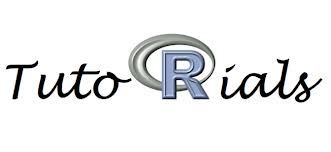# Tutorials- Introduction to R### Introduction to R

The tutorial is presented as a set of slides with the expectation that the reader will follow along typing the commands into R. The materials for the tutorial are:
In this tutorial, I give an overview of basic R concepts including:
• What is R?
• Getting R
• Resources for getting help within and about R
• R Data Structures
• Vectors
• Rectangular data--matrices and data frames
• Lists
• Factors
• Control structures, looping, and applying
• Introduction to functions

### Accomplishing Exome Sequencing Data Tasks with Bioconductor

This tutorial gives a few examples of how R and Bioconductor can be used to examine exome sequencing data. The pdf version of the tutorial is here. To get started, paste the following code into an R session. The BasicExomeExample package should be installed, also.
```
# install the BasicExomeExample package
install.packages('BasicExomeExample',

n.nci.nih.gov/~sdavis/software/R',
type='source'),type='source')
# the
contriburl=contrib.url('http://wats
ose are the vignette code examples, Sweave document, and PDF

a.R',
'BiocExomeData.R')
tv/~sdavis/BiocExomeData.Rnw',
'BiocExomeData.Rnw')

Data.pdf')

browseURL('http://watson.nci.nih.gov/~sdavis/BiocExom
e
```

### A Simple Data Integration Exercise Using Data from the TCGA Project

This tutorial is a simplistic introduction to data integration through experience and utilizes R and Bioconductor tools. Data from 45 TCGA glioblastoma multiforme patients are used to demonstrate how even a relatively simple analysis can lead to very interesting biological hypotheses. The tutorial combines:
• Gene expression data (Agilent)
• Gene copy number data (Agilent)
• DNA methyation data (Illumina array)

### Accessing Public Data using R and Bioconductor

This little tutorial describes the tools used to access public data from the NCBI Gene Expression Omnibus (GEO) and the Sequence Read Archive (SRA). Tools covered include GEOquery, GEOmetadb, and SRAdb. A short section on using the Integrative Genomic Viewer (IGV) from the Broad institute to visualize aligned reads is also included.
The goals of this tutorial are to:
• Understand the data relationships stored in the NCBI Gene Expression Omnibus
• Learn to use the GEOquery package to access data from NCBI GEO and understand the data structures used to capture these data in R
• Learn how the GEOmetadb package can be useful for finding data in NCBI GEO
• Use the SRAdb package for querying metadata from the NCBI SRA
• Access ENCODE metadata from R using RMySQL

### Introduction to Next-Generation Sequence Data Analysis Using Biowulf

The goals of this tutorial are to:
• Model a practical computational setup for next-gen sequence analysis including directory structure and installation and use of some common tools. Note: on helix and biowulf, some of these steps may not be necessary, as a large amount of software is already installed.
• Provide small example datasets that allow for a hands-on approach to sequence analysis.
• Promote experimentation and discussion regarding biological and technical questions related to handling sequence data.
Although this tutorial is designed for use on the NIH Biowulf cluster, the tutorial is general enough that any linux or even Mac OS system can probably be used to good effect. Go to the tutorial or go to the talk. Read  more